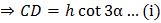# A tower subtends anglesrespectively at points, all lying on a horizontal line through the foot of the tower, thenis equal to a)b)c)d)## Question ID - 50766 :- A tower subtends anglesrespectively at points, all lying on a horizontal line through the foot of the tower, thenis equal to a)b)c)d)3537

(b)

InIn…(ii)

InFrom Eqs. (ii) and (iii),From Eqs. (i) and (ii),From Eqs. (iv) and (v),Next Question :
 A person standing on the bank of a river finds that the angle of elevation of the top of a tower on the opposite bank isthen which of the following statements is correct? a) Breadth of the river is twice the height of the tower b) Breadth of the river and the height of the tower are the same c) Breadth of the river is half of the height of the tower d) None of these